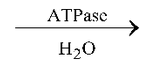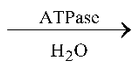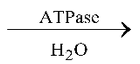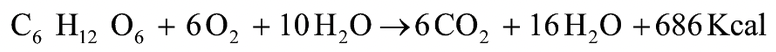# Introduction

## Cellular Respiration of Class 11

Respiration, term is coined by Dutrochet.

Cellular respiration is a Catabolic, exergonic (energy releasing), stepwise enzymatic breakdown of organic substrates like carbohydrates, fats and proteins to release energy in the form of ATP.

ATP is energy currency of the cell, was discovered by Lohman and ATP cycle (ATP–ADP system) was found by Lipmann.

ATPADP + ip + 8.9 Kcal (or 7.3 kcal according to old concept)

ADPAMP + ip + 6.5 Kcal

AMPA + Ribose + ip + 3.4 Kcal

General equation of cellular respiration is :(or 2880 KJ)

Efficiency of cellular respiration is calculated as 45% as the net gain of ATP molecules is 36 or 38.

Per O2 molecule energy obtained is 114.5 Kcal